# Polynomial Division Calculator

Created by Maciej Kowalski, PhD candidate
Reviewed by Dominik Czernia, PhD candidate and Jack Bowater
Last updated: Apr 06, 2022

Welcome to the polynomial division calculator, where we'll learn how to divide polynomials. You might remember that when we dealt with regular numbers, there was this handy algorithm called long division. In fact, here we have a similar one, polynomial long division, and it's just as easy. But don't worry, before we see how to do long division with polynomials, we'll slowly go through the basics and begin with dividing polynomials by monomials. Nice and easy, that's our motto.

So sit back, grab a cup of tea for the journey, and let's get to it!

## Polynomials, binomials, monomials

Do you remember the good ol' days when mathematics dealt only with numbers? Well, there were also squares and circles, but still, we described them using numbers. And then, some guy decided to get the alphabet involved and came up with algebra. Yeah, screw that guy too.

A polynomial is a sum of monomials. Now that is a helpful definition, isn't it? And we can go one step further: a monomial is a polynomial with only one summand. So, since we figured all of that out, let's jump to dividing polynomials using long division.

Oh, alright, we're not that evil. Let's go through it in detail.

A monomial is the product of numbers and variables with non-negative integer powers. This means that, for instance,

2x,

(-3) * z³ * 0.5,

πr²,

a²⁰²⁰,

½ * 0.7654321 * 1,

k * l * (-7x) * k

are all monomials. Observe that they don't necessarily have to contain variables.

The important thing here is that it cannot be the sum or difference of two expressions. (Note that above, the occasional minuses come from negative numbers, such as -3, and not from the subtraction operation.) Also, it cannot have any square roots or functions like sine or logarithm. Lastly, observe that we haven't written all of the above in their simplest form there is. For example, we can surely write ½ * 0.7654321 * 1 as a single number instead of that monstrosity.

So, coming back to the definition above, a polynomial is a sum of monomials. This means that, in particular, every monomial is a polynomial. Below we list a few other examples:

x + 2y,

a² + 2ab + b,

n³ - 0.7n + ⅜,

1 + 3 + x⁵ - x⁷ +19x⁵.

Lastly, a binomial is a polynomial with two summands. For instance, the first expression above is a binomial, while the others are not.

In general, a polynomial can have any number of variables. Today, however, we're going to focus on those that only have one variable, i.e., of the form

aₙxⁿ + aₙ₋₁xⁿ⁻¹ + ... + a₁x + a₀,

where aₙ, aₙ₋₁,..., a₁, a₀ are numbers, which we call coefficients.

By convention, the variable is usually denoted by x, but if you have any letter preferences, feel free to rebel and exchange it for whichever suits your needs. Just remember that this polynomial division calculator uses the x notation, and we will do so as well in the subsequent sections.

Now that we got familiar with the objects we're dealing with, we're one step closer to dividing polynomials with long division. However, let's start small and see the easiest case first - monomials.

## Dividing polynomials by monomials

Let's first get one thing straight - just as you can't divide a number by zero, you can't divide a polynomial by the zero polynomial, i.e., the polynomial with all coefficients equal to zero.

If, however, that is not the case, then everything works fine, and we can go on to the computations. Still, to get a good grasp of the topic, before we see how to divide polynomials in general we'll study the simplest case: dividing polynomials by monomials. In other words, we want to find P(x) / Q(x) when Q(x) = bₖxᵏ for some non-negative integer k.

As a rule, P(x) can be anything, so let's denote it as we did in :

P(x) = aₙxⁿ + aₙ₋₁xⁿ⁻¹ + ... + a₁x + a₀.

The trick here is that we can now divide the polynomials by taking each summand of P(x) separately. In other words,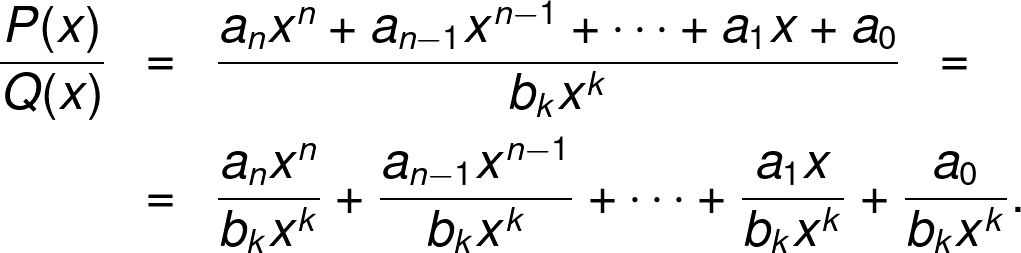Now, according to the properties of the exponent, we know that

xˢ / xᵗ = xˢ⁻ᵗ.

But we don't want any negative powers in the variables! We like polynomials, after all. Therefore, we'll group all the terms of P(x) with smaller exponents in x and write them as the (non-divisible) rest of the polynomial division.

All in all, we get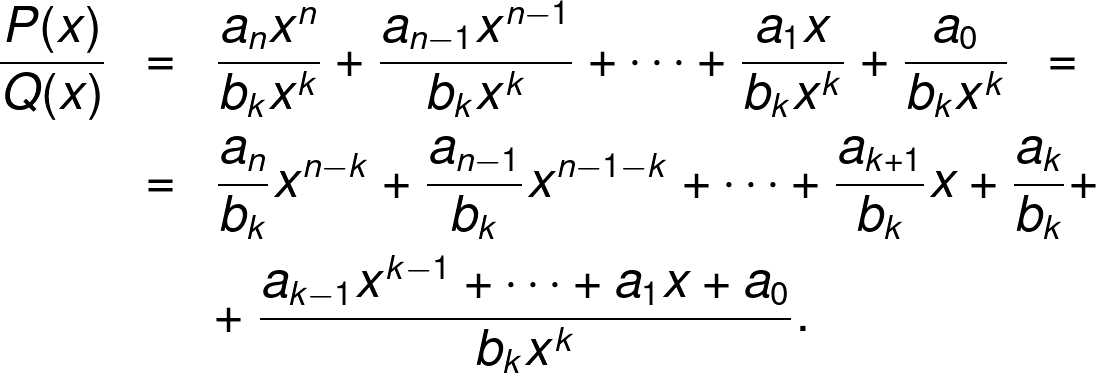Not too bad, was it? It may not be a walk in the park, but it's not rocket science either. Now it's only a matter of generalizing it so we can divide polynomials by more than just monomials.

Shall we?

## How to divide polynomials?

The basic idea behind the polynomial division algorithm is:

1. Take the polynomial P(x) that you want to divide by Q(x). Unless the degree of P(x) is smaller than that of Q(x), proceed. Otherwise, P(x) is the rest of the polynomial division.
2. Look at the maximal (in terms of the exponent of x) summand in P(x) and divide it by the maximal summand in Q(x). The result is a (monomial) summand of your quotient.
3. Multiply that monomial by Q(x) and subtract the result from P(x).
4. The difference you obtain is of a smaller degree than P(x). Let it be your new dividend (your new P(x)), and keep Q(x) as it was.
5. Repeat.

In essence, what it all boils down to is that you only take into account the largest (in terms of the exponent of x) summands of the dividend P(x) and make that summand vanish using Q(x). You then end up with something smaller, and you repeat the process until this smaller is too small to handle.

We'll study in detail how to do long division with polynomials in , but for now, let's see a sketch of the algorithm at work.

Say that you have a polynomial P(x) = aₙxⁿ + aₙ₋₁xⁿ⁻¹ + ... + a₁x + a₀ that you want to divide by Q(x) = bₖxᵏ + bₖ₋₁xᵏ⁻¹ + ... + b₁x + b₀, and assume that n > k so that there's actually some polynomial division to do. Then, we look at the first summands, aₙxⁿ and bₖxᵏ (of course, we assume that aₙ and bₖ are non-zero), and divide one by the other:

aₙxⁿ

bₖxᵏ
=
aₙ

bₖ
xⁿ⁻ᵏ

This result goes into the quotient. Next, we do the subtraction:

P(x) - (aₙ/bₖ)xⁿ⁻ᵏ * Q(x).

This will give us a new polynomial with brand new coefficients. What is important about it is that its degree is strictly smaller than the degree of P(x) because (aₙ/bₖ)xⁿ⁻ᵏ has been chosen precisely so that it kills the aₙxⁿ in P(x). Then, we take this new polynomial and repeat the whole thing.

We now know how to divide polynomials or, at least, the idea behind the algorithm. It's now time to introduce the polynomial long division, an easy tool to keep track of every step in the process.

Excited? We know we are! Let's not waste a second longer and learn all about dividing polynomials using long division!

## How to do long division with polynomials?

Let's get back to the notation we've used in : we want to divide P(x) = aₙxⁿ + aₙ₋₁xⁿ⁻¹ + ... + a₁x + a₀ by Q(x) = bₖxᵏ + bₖ₋₁xᵏ⁻¹ + ... + b₁x + b₀. Let's write the basic outline of this operation similarly to how we do with long division of numbers: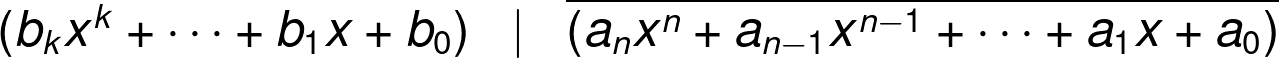From , we know that the first step in the polynomial long division gives us the summand (aₙ/bₖ)xⁿ⁻ᵏ in the quotient. We write it above the line: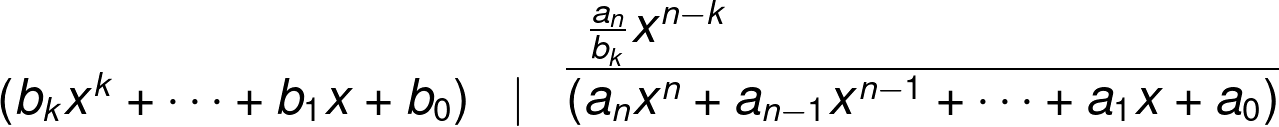and we write under (aₙxⁿ + aₙ₋₁xⁿ⁻¹ + ... + a₁x + a₀) what we will subtract from it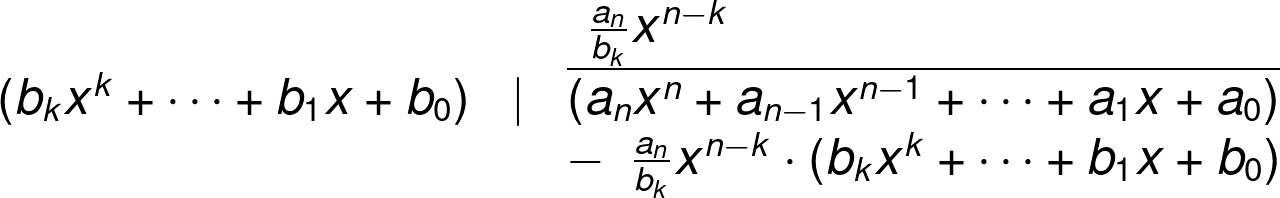Now, we do the subtraction: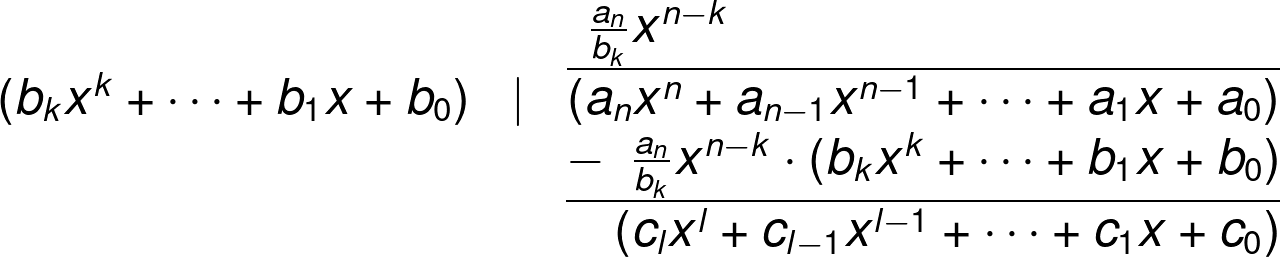for appropriate cᵢ-s and l < n. Then, as long as l is not smaller than k, we repeat this process for the new polynomial: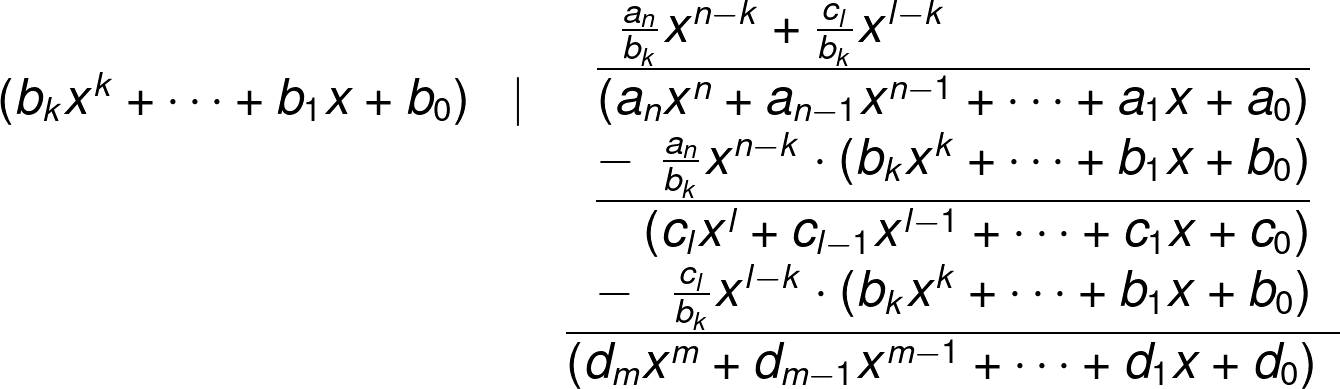for appropriate dᵢ's and m < l.

We continue this process as long as we continue to obtain polynomials with a degree of at least k. Once we go below, we finish the polynomial long division. All in all, we should get something of the form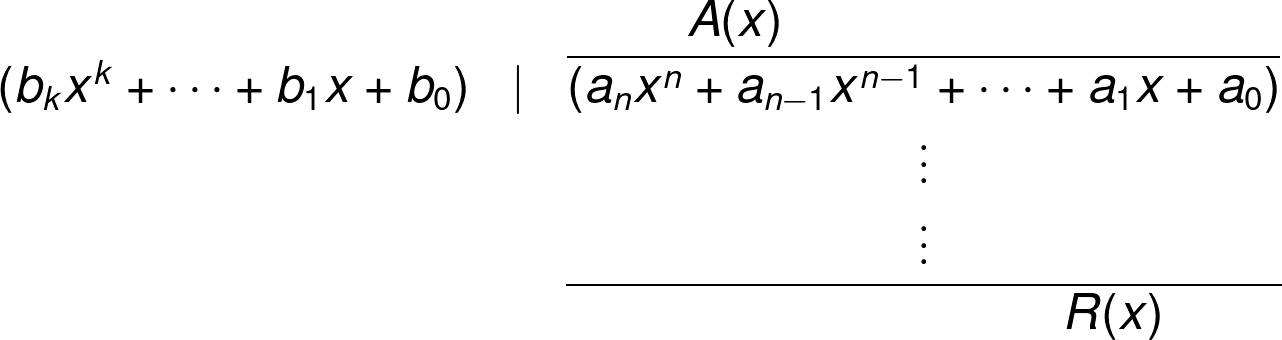for some polynomials A(x) and R(x) with R(x) being a smaller degree than Q(x). This translates to

P(x)

Q(x)
= A(x) +
R(x)

Q(x)

Phew, that was a long one, wasn't it? It's high time we reward all this time spent on theory\ by a nice example that actually has numbers in it. With that, dividing polynomials using long division will become so much clearer!

## Example: using the polynomial division calculator

The formula for success is

P(x) = x⁴ - 27x³ + 239x² - 753x + 540.

At least, that's what the fortune cookie told you at the restaurant you're visiting. Apparently, every root (i.e., a value of x for which the expression is equal to zero) of P(x) shows you how many years until you'll have the best year of your life. For instance, if 7 were such a root, then in seven years time, you should have a pretty awesome year.

Seems legit, right?

You throw in a good tip and run home to study your precious formula. But how do we do it? With the help of , of course!

Unfortunately, our formula is of degree 4 (the highest power of x that appears in the polynomial). Contrary to, say, quadratic equations, this one doesn't have a nice way of finding its roots. Fortunately for us, there is another way!

Given a polynomial, a number a is its root if and only if the polynomial is divisible by (x - a) (this, in fact, is a very non-trivial statement called Bézout's theorem). Therefore, we can make use of the polynomial division calculator to determine when your amazing year will be.

However, as we've already mentioned, we don't know the roots. So why don't we try a different approach: let's see if next year is going to be successful! In other words, we'll check if 1 is a root, or, equivalently, if P(x) is divisible by (x - 1).

Before we begin dividing the polynomials using long division, let's take a minute to see how easily the polynomial division calculator deals with such problems.

We wish to divide P(x) = x⁴ - 27x³ + 239x² - 753x + 540 by Q(x) = x - 1. All the calculator needs from us is to input this data. We begin by telling it the degrees of both polynomials: in our case, it's 4 for P(x) and 1 for Q(x). We choose the correct options from the lists for each under "Degree" in the corresponding calculator sections.

Once this is done, the polynomial division calculator will show us the notation for P(x) and Q(x), i.e., that

P(x) = a₄x⁴ + a₃x³ + a₂x² + a₁x + a₀,

and

Q(x) = b₁x + b₀.

This means that the consecutive coefficients (from the largest exponent down) of P(x) are a₄, a₃, a₂, a₁, and a₀. Looking back at the success formula, we input

a₄ = 1, a₃ = -27, a₂ = 239, a₁ = -753, a₀ = 540.

(Remember that when we have no number in front of a variable, like with x⁴ in P(x), then this means that the coefficient is 1.)

Similarly for Q(x), we input

b₁ = 1, b₀ = -1.

The moment we write the last number, the polynomial division calculator will spit out the answer. But let's not spoil it for ourselves just yet! How about we grab a piece of paper and do the polynomial long division ourselves?

If we recall the , we'll know to begin with an outline:

(x - 1) | (x⁴ - 27x³ + 239x² - 753x + 540)

Now, we take the first summand of P(x), which is x⁴, and divide it by the first summand of Q(x), i.e., by x:

x⁴ / x = x³.

This gives us the first element of the quotient, which we write above the line.

x³

(x - 1) | (x⁴ - 27x³ + 239x² - 753x + 540)

Next, we multiply the x³ by the divisor Q(x), and subtract it from the dividend P(x):

x³

(x - 1) | (x⁴ - 27x³ + 239x² - 753x + 540)
-    x³ * (x - 1)

-26x³ + 239x² - 753x + 540

Just this one time, let's study in detail how the bottom line came to be:

(x⁴ - 27x³ + 239x² - 753x + 540) - x³ * (x - 1) =

= x⁴ - 27x³ + 239x² - 753x + 540 - x⁴ + x³ =

= -26x³ + 239x² - 753x + 540.

Next, we take the first summand of the polynomial we just got, which is -26x³, and divide it by the first summand of Q(x), i.e., by x:

-26x³ / x = -26x².

This monomial goes to the top line, where we write our quotient, and we repeat the other steps as well:

x³ - 26x²

(x - 1) | (x⁴ - 27x³ + 239x² - 753x + 540)
-    x³ * (x - 1)

-26x³ + 239x² - 753x + 540
-    -26x² * (x - 1)

213x² - 753x + 540

Now, we have

213x² / x = 213x,

which gives

x³ - 26x² + 213x

(x - 1) | (x⁴ - 27x³ + 239x² - 753x + 540)
-    x³ * (x - 1)

-26x³ + 239x² - 753x + 540
-    -26x² * (x - 1)

213x² - 753x + 540
-    213x * (x - 1)

-540x + 540

Lastly,

-540x / x = -540,

and

x³ - 26x² + 213x - 540

(x - 1) | (x⁴ - 27x³ + 239x² - 753x + 540)
-    x³ * (x - 1)

-26x³ + 239x² - 753x + 540
-    -26x² * (x - 1)

213x² - 753x + 540
-    213x * (x - 1)

-540x + 540
-    -540 * (x - 1)

0

Great, we got 0! This means that

x⁴ - 27x³ + 239x² - 753x + 540

x - 1
=
 = x³ - 26x² + 213x - 540

Nothing else, no remainder. This means that the P(x) is indeed divisible by x - 1, which means that 1 is a root of P(x). So next year is really going to be successful, how wonderful! And oh, with what's been happening recently, we do appreciate some good news, don't we?

Maciej Kowalski, PhD candidate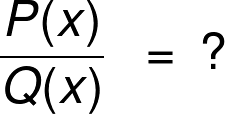Polynomial P(x)
Degree
2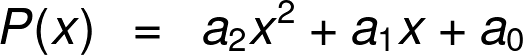a₂
a₁
a₀
Polynomial Q(x)
Degree
1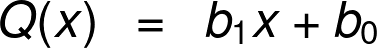b₁
b₀
People also viewed…

### Alien civilization

The alien civilization calculator explores the existence of extraterrestrial civilizations by comparing two models: the Drake equation and the Astrobiological Copernican Limits👽

### Cylinder volume gallons

Use this cylinder volume gallons calculator to determine cylinder volume in gallons.

### Square Pyramid

Using the square pyramid calculator, find the surface area and volume of a square pyramid.

### Steps to calories

Steps to calories calculator helps you to estimate the total amount to calories burned while walking.